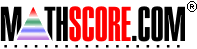Math Practice Online > free > lessons > Florida > 6th grade > Probability 2

## Probability 2

This topic covers harder probability problems. You may need pencil and paper.

 Sample Problems for Probability 2 Lesson for Probability 2

### This topic aligns to the following state standards

Grade 7: Dat 3. Calculates simple mathematical probabilities for independent and dependent events.
Grade 8: Dat 2. Calculates simple mathematical probabilities for independent and dependent events.
Grade 9: Dat 2. determines the probability for simple and compound events as well as independent and dependent events
Grade 6: Dat 1. Examines and describes situations that include finding the odds for and against a specified outcome.
Grade 7: Dat 1. Computes the mathematical odds for and against a specified outcome in given real-world experiments.
Grade 8: Dat 1. Predicts the mathematical odds for and against a specified outcome in a given real-world situation.

Copyright Accurate Learning Systems Corporation 2008.